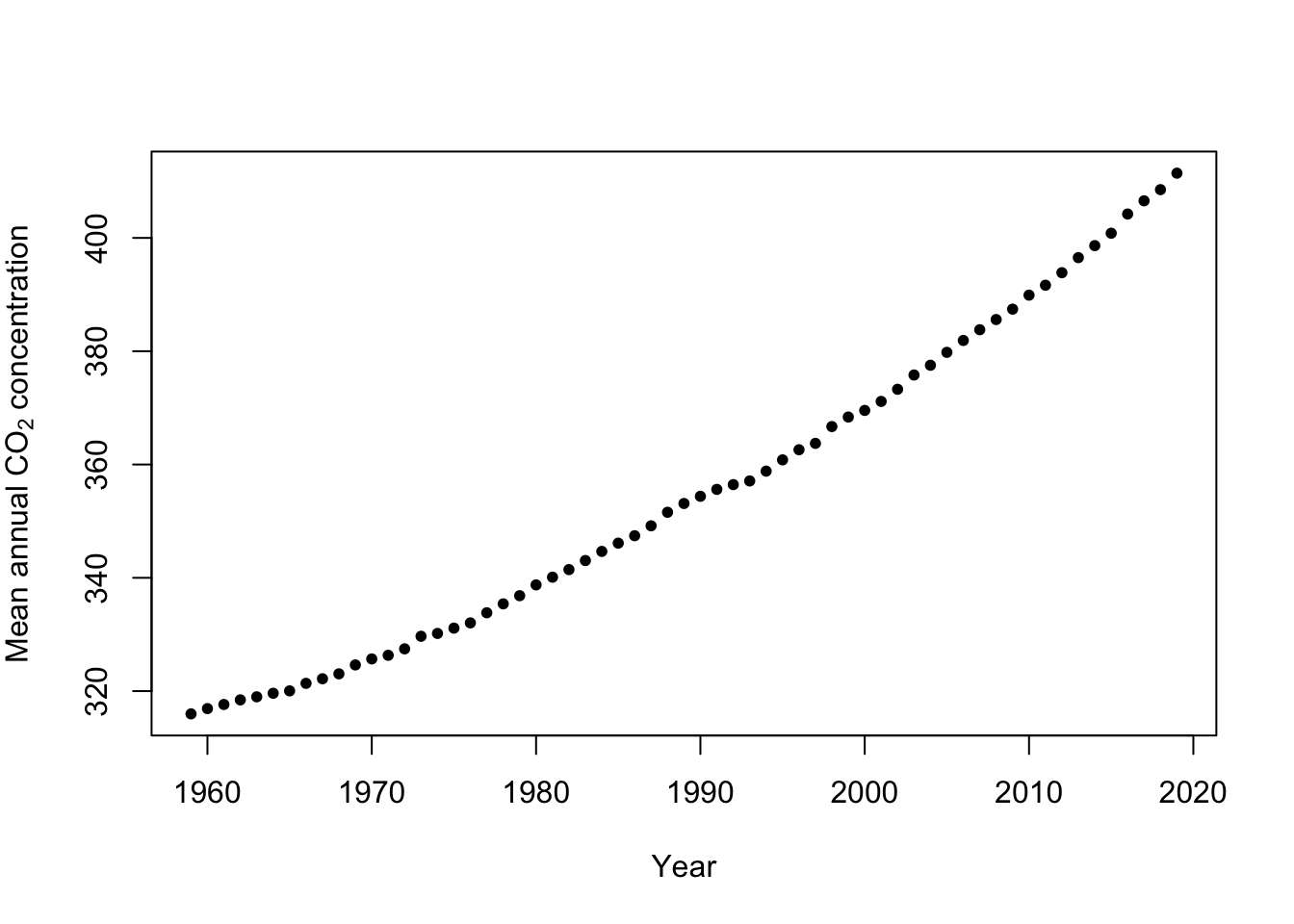# 10 September 17

## 10.1 Announcements

• No new announcements

## 10.2 Extreme precipitation in Kansas

• What we will need to learn
• How to use R as a geographic information system
• New general tools from statistics
• Gaussian process
• Metropolis and Metropolis–Hastings algorithms
• Gibbs sampler
• How to use the hierarchical modeling framework to describe Kriging
• Hierarchical Bayesian model vs. “empirical” hierarchical model
• Specialized language used in spatial statistics (e.g., range, nugget, variogram)

## 10.3 Gibbs sampler

• History
• Stochastic Relaxation, Gibbs Distributions, and the Bayesian Restoration of Images (Geman and Geman 1984))
• Sampling-Based Approaches to Calculating Marginal Densities (Gelfand and Smith 1990)
• The gibbs sampler is responsible for the recent “Bayesian revolution”
• Gibbs sampler
• See pg. 169 in book.
• In many cases the distribution we want to sample from is multivariate
• If we can sample from the univariate full-conditional distribution for each random variable of a multivariate distribution, then we can use a Gibbs sampler to obtain dependent sample from the multivariate distribution

### 10.3.1 Gibbs sampler: simple example

$\boldsymbol{\theta}\sim\text{N}\Bigg(\mathbf{0},\bigg[\begin{array}{cc} 1 & \rho\\ \rho\ & 1 \end{array}\bigg]\Bigg)$ where $$\boldsymbol{\theta}\equiv(\theta_{1},\theta_{2})'$$. Suppose we didn’t know how to sample from the multivariate normal distribution, but we could obtain the conditional distributions $$[\theta_{1}|\theta_{2}]$$ and $$[\theta_{2}|\theta_{1}]$$ which are $\theta_{1}|\theta_{2}\sim\text{N}(\rho\theta_{2},1-\rho^{2})$ $\theta_{2}|\theta_{1}\sim\text{N}(\rho\theta_{1},1-\rho^{2})\,.$ It turns out that if we iteratively sample from $$[\theta_{1}|\theta_{2}]$$ and then $$[\theta_{2}|\theta_{1}]$$ (or the other way around) we will obtain samples from $$[\boldsymbol{\theta}]$$.

### 10.3.2 Gibbs sampler: Bayesian linear model example

• The model
• Distribution of the process: “$$\mathbf{y}$$ given $$\boldsymbol{\beta}$$ and $$\sigma_{\varepsilon}^{2}$$$\mathbf{y}|\boldsymbol{\beta},\sigma_{\varepsilon}^{2}\sim\text{N}(\mathbf{X}\boldsymbol{\beta},\sigma_{\varepsilon}^{2}\mathbf{I})$ This is also sometimes called the process model.
• Distribution of the parameters: $$\boldsymbol{\beta}$$ and $$\sigma_{\varepsilon}^{2}$$ $\boldsymbol{\beta}\sim\text{N}(\mathbf{0},\sigma_{\beta}^{2}\mathbf{I})$ $\sigma_{\varepsilon}^{2}\sim\text{inverse gamma}(q,r)$
• Computations and statistical inference
• Using Bayes rule (Bayes 1763) we can obtain the joint posterior distribution $[\boldsymbol{\beta},\sigma_{\varepsilon}^{2}|\mathbf{y}]=\frac{[\mathbf{y}|\boldsymbol{\beta},\sigma_{\varepsilon}^{2}][\boldsymbol{\beta}][\sigma_{\varepsilon}^{2}]}{\int\int [\mathbf{y}|\boldsymbol{\beta},\sigma_{\varepsilon}^{2}][\boldsymbol{\beta}][\sigma_{\varepsilon}^{2}]d\boldsymbol{\beta}d\sigma_{\varepsilon}^{2}}$
• Statistical inference about a paramters is obtained from the marginal posterior distributions $[\boldsymbol{\beta}|\mathbf{y}]=\int[\boldsymbol{\beta},\sigma_{\varepsilon}^{2}|\mathbf{y}]d\sigma_{\varepsilon}^{2}$ $[\sigma_{\varepsilon}^{2}|\mathbf{y}]=\int[\boldsymbol{\beta},\sigma_{\varepsilon}^{2}|\mathbf{y}]d\boldsymbol{\beta}$
• In practice it is difficult to find closed-form solutions for the marginal posterior distributions, but easy to find closed-form solutions for the conditional posterior distribtuions.
• Full-conditional distribtuions: $$\boldsymbol{\beta}$$ and $$\sigma_{\varepsilon}^{2}$$ $\boldsymbol{\beta}|\sigma_{\varepsilon}^{2},\mathbf{y}\sim\text{N}\bigg{(}\big{(}\mathbf{X}^{\prime}\mathbf{X}+\frac{1}{\sigma_{\beta}^{2}}\mathbf{I}\big{)}^{-1}\mathbf{X}^{\prime}\mathbf{y},\sigma_{\varepsilon}^{2}\big{(}\mathbf{X}^{\prime}\mathbf{X}+\frac{1}{\sigma_{\beta}^{2}}\mathbf{I}\big{)}^{-1}\bigg{)}$ $\sigma_{\varepsilon}^{2}|\mathbf{\boldsymbol{\beta}},\mathbf{y}\sim\text{inverse gamma}\left(q+\frac{n}{2},(r+\frac{1}{2}(\mathbf{y}-\mathbf{X}\boldsymbol{\beta})^{'}(\mathbf{y}-\mathbf{X}\boldsymbol{\beta}))^-1\right)$
• Gibbs sampler for the Bayesian linear model
• Write out on the whiteboard
• Example: Mauna Loa Observatory carbon dioxide data

url <- "ftp://aftp.cmdl.noaa.gov/products/trends/co2/co2_annmean_mlo.txt"
colnames(df) <- c("year", "meanCO2", "unc")
plot(x = df$year, y = df$meanCO2, xlab = "Year", ylab = expression("Mean annual " *
CO * " concentration"), col = "black", pch = 20)• Gibb sampler
# Preliminary steps
library(mvnfast)  # Required package to sample from multivariate normal distribution

y <- df\$meanCO2  # Response variable
X <- model.matrix(~year, data = df)  # Design matrix
n <- length(y)  # Number of observations
p <- 2  # Dimensions of beta

m.draws <- 5000  #Number of MCMC samples to draw

samples <- as.data.frame(matrix(, m.draws, 3))  # Samples of the parameters we will save
colnames(samples) <- c(colnames(X), "sigma2")

sigma2.e <- 1  # Starting values for sigma2

sigma2.beta <- 10^9  #Prior variance for beta
q <- 2.01  #Inverse gamma prior with E() = 1/(r*(q-1)) and Var()= 1/(r^2*(q-1)^2*(q-2))
r <- 1

# MCMC algorithm
for (m in 1:m.draws) {
# Sample beta from full-conditional distribution
H <- solve(t(X) %*% X + diag(1/sigma2.beta, p))
h <- t(X) %*% y
beta <- t(rmvn(1, H %*% h, sigma2.e * H))

# Sample sigma from full-conditional distribution
q.temp <- q + n/2
r.temp <- (1/r + t(y - X %*% beta) %*% (y - X %*% beta)/2)^-1
sigma2.e <- 1/rgamma(1, q.temp, , r.temp)

# Save samples of beta and sigma
samples[m, ] <- c(beta, sigma2.e)
}

# Look a histograms of posterior distributions
par(mfrow = c(3, 1), mar = c(5, 6, 1, 1))
hist(samples[, 1], xlab = expression(beta * "|" * bold(y)), ylab = expression("[" *
beta * "|" * bold(y) * "]"), freq = FALSE, col = "grey", main = "", breaks = 30)
hist(samples[, 2], xlab = expression(beta * "|" * bold(y)), ylab = expression("[" *
beta * "|" * bold(y) * "]"), freq = FALSE, col = "grey", main = "", breaks = 30)
hist(samples[, 3], xlab = expression(sigma^2 * "|" * bold(y)), ylab = expression("[" *
sigma^2 * "|" * bold(y) * "]"), freq = FALSE, col = "grey", main = "", breaks = 30)

# Mean of the posterior distribution
colMeans(samples[, 1:2])

# Compare to results obtained from lm function
m1 <- lm(y ~ X - 1)
coef(m1)